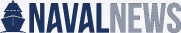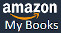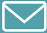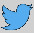The more torpedoes and missiles a submarine carries, the more targets it can attack. There are many other variables at play (context is everything), but the maximum weapons load of a submarine is a real consideration. This ranking is compiled from my submarine database (see the Covert Shores recognition book HERE). I have calculated it based on total number of torpedo tubes (assuming that they can be used to carry a torpedo), plus the maximum number of torpedo racks in the torpedo room, plus any VLS or slanted lanch tubes.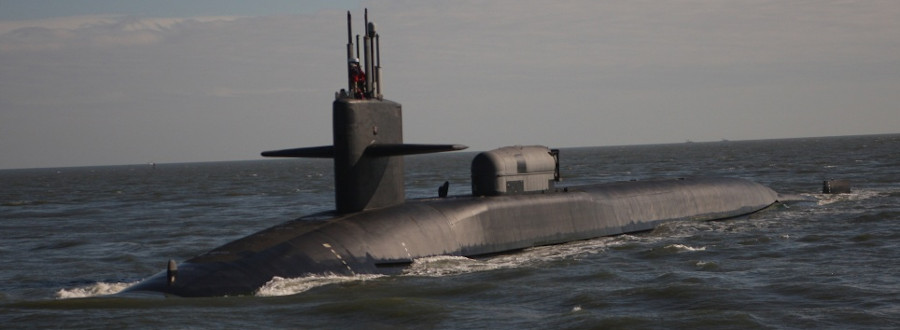The US Navy's Ohio Class SSGN is the undisputed king of weapons load. US Navy photo of USS Georgia (SSGN 729)(PO1 Kimberly Clifford)

The history and evolution of the torpedo room is really interesting. And generally not well understood by the general public. So it is worth a whole article in its own right,...

The ranking assumes that every possible 'slot' is occupied. This is unrealistic in many cases but is the fairest way to compare them. I have assumed full-sized weapons, except in submarines specifically designed to carry a smaller weapon as part of their main arsenal (e.g. Swedish submarines using 400mm weapons). I have excluded mines carried externally.

Get The essential guide to World Submarines
This Covert Shores Recognition Guide Covers over 80 classes of submarines including all types currently in service with World Navies.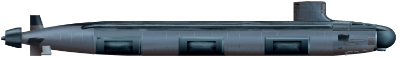Check it out on Amazon

Finally, ballistic missile submarines (SSBN / SSB / SSBA) are excluded, as are a handful of special mission submarines (mostly unarmed anyway) and dedicated test submarines.

RANKINGs
Rank, Class, Country of Design, Torpedo Tubes, Torpedo Room, VLS, Total (notes)

1. Ohio (SSGN), United States. 4 + 18 + 154 = 176
2. SEVERODVINSK-II, Russia. 10 + 30 + 32 = 72
3. OSCAR-II, Russia. 6 + 22 + 24 = 52 (Will increase to 100 with 'AM' modernization)
4. Seawolf Class, United States. 8 + 42 + 0 = 50
5. AKULA Class, Russia. 8 + 32 + 0 = 40 (Baseline. Excludes decoy tubes.)
5. SIERRA Class, Russia. 8 + 32 + 0 = 40 (SIERRA-I / II)
7. Astute Class, United Kingdom. 6 + 32 + 0 = 38
7. Virginia Class (Block IV), United States. 4 + 22 + 12 = 38
9. Improved Los-Angeles Class, United States. 4 + 21 + 12 = 37
10. Trafalgar Class, United Kingdom. 5 + 25 + 0 = 30
---------------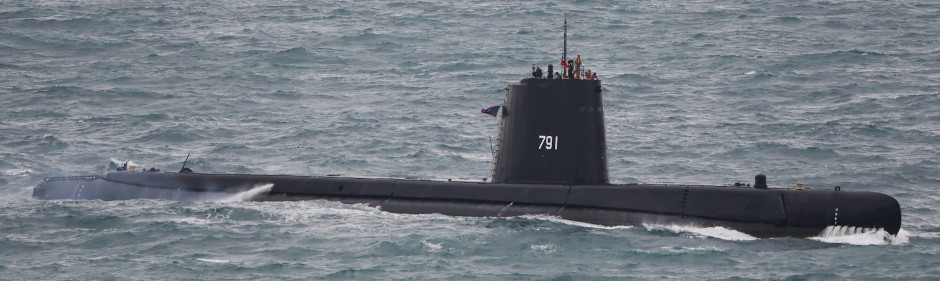The highest ranking non-nuclear submarine is also the oldest submarine in service in the world. Taiwan still operates two World War Two vintage US Navy Fleet Submarines as the Hai Shih class. They can carry 28 torpedoes and Harpoon missiles (sources vary).

Original artwork, CLICK IMAGE FOR HIGH-RESOLUTION: (on NavalNews.com)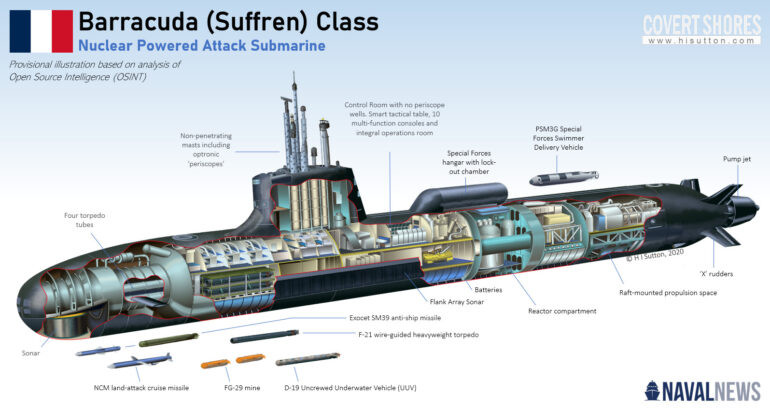The French Navy's new Suffren Class SSN can carry a theoretical maximum load of 24 torpedoes. This is significantly more than the preceding Rubis Class (14) but fewer than other Western or Russian SSNs.

11. Hai Shih (GUPPY-II) Class, United States. 10 + 18 + 0 = 28 (Includes aft torpedo room, 24 & 26 also quoted)
12. Suffren Class, France. 4 + 20 + 0 = 24
12. VICTOR-III Class, Russia. 6 + 18 + 0 = 24
14. Collins Class, Australia. 6 + 16 + 0 = 22
14. Type-093A Shang-II, China. 6 + 16 + 0 = 22
14. Gotland Class, Sweden. 8 + 14 + 0 = 22 (Includes 400mm weapons)
14. Santa Cruz Class, Germany. 6 + 16 + 0 = 22
18. Soryu Class, Japan. 6 + 15 + 0 = 21
19. Dosan Ahn Changho Class, South Korea. 6 + 8 + 6 = 20
19. A26 Blekinge Class, Sweden. 4 + 16 + 0 = 20 (Includes 400mm weapons, excludes Flex tube)
19. Walrus Class, Netherlands. 4 + 16 + 0 = 20
19. Oyashio Class, Japan. 6 + 14 + 0 = 20
19. Hai Lung Class, Netherlands. 6 + 14 + 0 = 20
---------------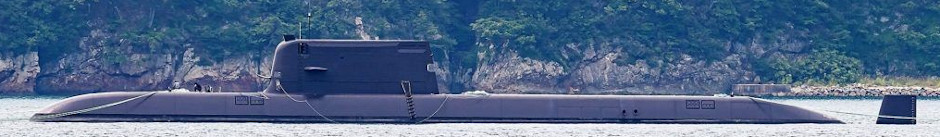South Korea's Dosan Ahn Changho Class (aka KSS-III) is the first modern non-nuclear submarine with a VLS for cruise missiles. Its internal weapons stowage is modest however.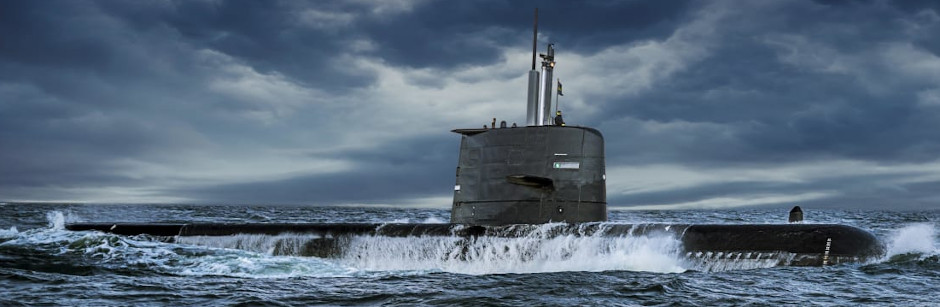Although not large boats, Swedish submarines have a trick up their sleeve which results in high torpedo load-outs. They are designed to carry both regular 533mm heavyweight torpedoes and smaller 400mm weapons. The 400mm type can be carried and launched in tandem, thus doubling their number. (Saab photo)

24. Kilo Class, Russia. 6 + 12 + 0 = 18
24. Victoria (Upholder) Class, United Kingdom. 6 + 12 + 0 = 18
24. Scorpène Class, France. 6 + 12 + 0 = 18
24. Isaac Peral Class, Spain. 6 + 12 + 0 = 18
24. Type-091 Han Class, China. 6 + 12 + 0 = 18 (Est.)
24. LADA Class, Russia. 6 + 12 + 0 = 18
24. Södermanland class, Sweden. 12 + 6 + 0 = 18 (Includes 400mm weapons)
31. Type 039A Yuan Class, China. 6 + 10 + 0 = 16 (Est.)
31. Type-039 Song Class, China. 6 + 10 + 0 = 16 (Est.)
31. Type-214 Class, Germany. 8 + 8 + 0 = 16
31. Type-218SG Class, Germany. 8 + 8 + 0 = 16
31. Agosta-90B Class, France. 4 + 12 + 0 = 16
31. Dolphin Class, Germany. 10 + 6 + 0 = 16 (Dolphin I / II)
37. Rubis Class, France. 4 + 10 + 0 = 14
37. Type-209 Class, Germany. 8 + 6 + 0 = 14
37. Ula Class, Germany. 8 + 6 + 0 = 14
37. Type-035 Ming Class, China. 8 + 6 + 0 = 14
37. Romeo Class, Russia. 8 + 6 + 0 = 14
42. Type-212A Class, Germany. 6 + 6 + 0 = 12
42. Sauro Class, Italy. 6 + 6 + 0 = 12
42. Sjöormen Class, Sweden. 6 + 6 + 0 = 12 (Includes 400mm weapons)
---------------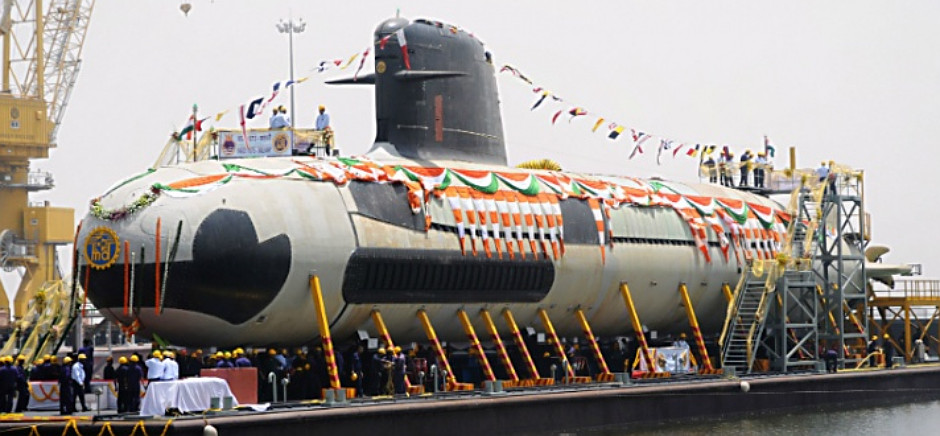The French Scorpene class design is typical of modern diesel-electric submarines with a maximum load of 18 weapons. 18 seems to be the sweet spot, at least for now.

45. Type-206 Class, Germany. 8 + 0 + 0 = 8
45. Kobben Class, Germany. 8 + 0 + 0 = 8
47. Fateh Class, Iran. 4 + 2 + 0 = 6 (Est. possible reloads)
---------------

Original artwork based on photo analysis - CLICK IMAGE FOR HIGH-RESOLUTION: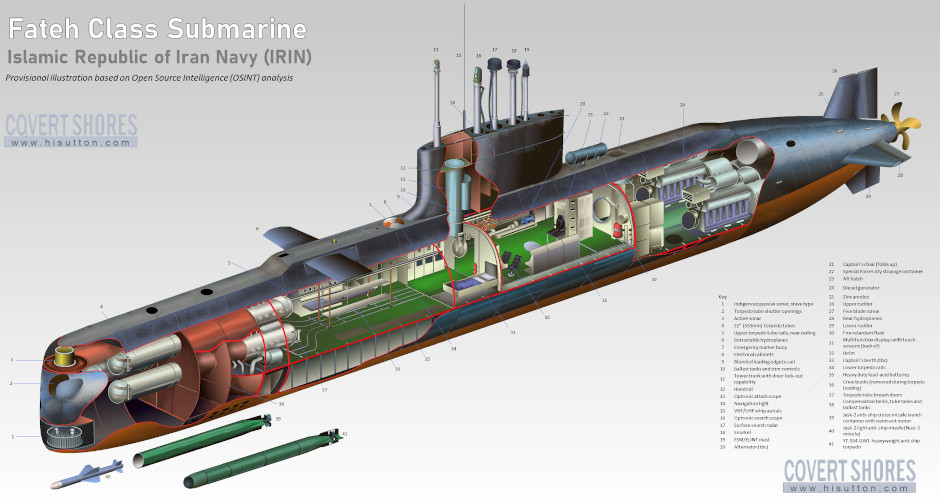Some smaller submarines, such as the Iranian Fateh Class, have limited space for internal stowage of torpedoes. It is similar to the Cold War vintage German designed Type-206 and Kobben classes which had a lot of torpedo tubes but no reloads.

48. HDS-400, South Korea. 4 + 0 + 0 = 4 (Likely includes smaller diameter weapons)
49. SX-756/K Class, Italy. 2 + 0 + 0 = 2
49. MG-110 Class, Italy. 2 + 0 + 0 = 2
49. Yugo Class, North Korea. 2 + 0 + 0 = 2
49. Sang-0 Class, North Korea. 2 + 0 + 0 = 2
49. Yono Class, North Korea. 2 + 0 + 0 = 2 (Also Ghadir Class in Iran)

Related articles (Full index of popular Covert Shores articles)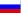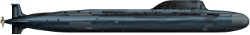Project 885 'YASEN' (Severodvinsk Class) SSGN. w/Cutaway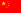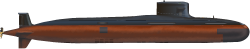Chinese Type-093 Shang Class Submarine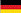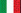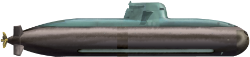Type-212 AIP submarine w/Cutaway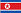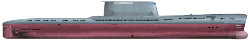North Korean Modified-Romeo